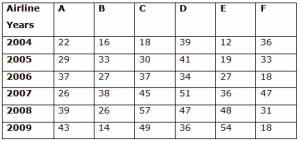## Data Interpretation For SBI PO : Set – 30

D.1-4) These questions are based on the following information:

The legislative council in a state consists of members of five parties  BJP, Congress, JD, SP and CPI. Each of the members has exactly one profession  Doctor, Lawyer, Actor, Teacher or Engineer and no member belongs to more than one party.1) The number of teachers among the members of the council is the same as the number of members of which party?

a) BJP

b) Congress

c) CPI

d) SP

e) None of these

d)2) The number of members of which party is double the number of members who are doctors?

a) BJP

b) CPI

c) JD

d) SP

e) None of these

a)3) If the strength of the Congress party in the council is 240, then what is the number of council members who are engineers?

a) 120

b) 150

c) 225

d) 300

e) None of these

d)

Total congress party members are 240 in this 25% are engineers.But compare to their professions 20% of engineers are only belong to congress , by this4) If there are a total of 150 actors in the council, then what is the total strength of the council?

a) 450

b) 500

c) 550

d) 600

e) None of these

e)D.5-10) Study the following table carefully and answer the questions given below it.

Number of passengers (in Thousands) booking tickets in six different airlines in six years)5) What is the average number of passengers booking tickets in year 2006 in all the airlines together?

a) 30,000

b) 10,000

c) 18,000

d) 27,000

e) None of these

a)

Average Number of Passengers booking tickets in year 2006 = 37000+27000+37000+34000+27000+18000=180/6=30000

6) What is the average number of passengers booking tickets in airlines F in all the years together?

a) 3, 05, 000

b) 35,000

c) 30,500

d) 3,15,00

e) None of these

c)

Average Number of Passenger booking tickets in airlines  F in all years =

36000+33000+18000+47000+31000+18000=183000/6=30,500

7) What is the respective ratio between the passengers booking tickets in airlines A in year 2008 and in airline E in year 2007?

a) 12: 13

b) 13: 12

c) 3 : 4

d) 4 : 3

e) None of these

b)

Passengers booking tickets in airlines A in 2008  : Passenger booking tickets in airlines E in 2007

39000 : 36000

13:12

8) What is the total number of passengers booking tickets in airlines F and B together in the year 2009 and passengers booking tickets in airline A and D in year 2008 together?

a) 1, 80000

b) 1, 15,000

c) 11,800

d) 11,080

e) None of these

e)

Total Number airlines F and B together in the year 2009 = 36000+18000 = 54000

Total Number of airlines A and D together in 2008 = 39000+57000 = 96000

Total = 54000+96000= 150000

9) What is the difference between total number of passengers booking tickets in airlines
E and F together in the year 2008 and passengers booking tickets in airline A and C in year 2009 together?

a) 12000

b) 13000

c) 12500

d) 14000

e) None of these

b)

Total Number of passengers booking tickets in airlines E and F in year 2008  = 48000+31000 = 79000

Total Number of passenger booking tickets in airlines A and C in year 2009 = 43000+ 49000 = 92000

Difference = 92000 – 79000 = 13000

10) What is the average number of passengers booking tickets in airlines  E in all the years together?

a) 65433

b) 65334

c) 65000

d) 65390

e) 67000